# Upcoming ClassesPage 2

#### WATCH ALL SLIDES

Dance is artistic motion of the human body.

Ballet

Acrobats

(Cirque du Soleil)

Break

Dancing

Athletics

Tommie Smith & John Carlos

Mexico City 1968 Olympics

Slide 10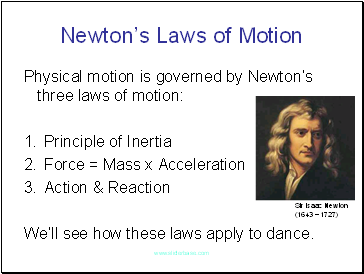## Newton’s Laws of Motion

Physical motion is governed by Newton’s three laws of motion:

Principle of Inertia

Force = Mass x Acceleration

Action & Reaction

We’ll see how these laws apply to dance.

Sir Isaac Newton

(1643 – 1727)

Slide 11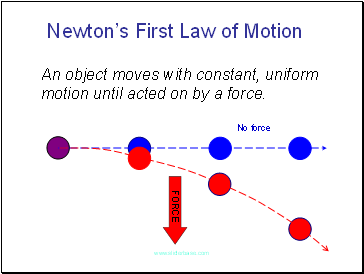Newton’s First Law of Motion

An object moves with constant, uniform motion until acted on by a force.

FORCE

No force

Slide 12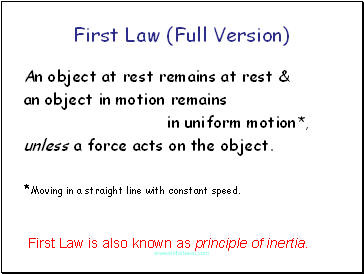First Law (Full Version)

An object at rest remains at rest &

an object in motion remains

in uniform motion*,

unless a force acts on the object.

*Moving in a straight line with constant speed.

First Law is also known as principle of inertia.

Slide 13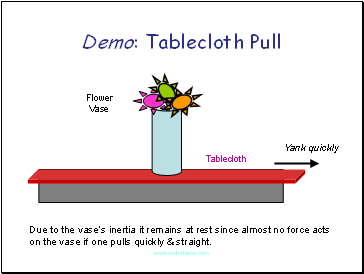Demo: Tablecloth Pull

Tablecloth

Flower

Vase

Yank quickly

Due to the vase’s inertia it remains at rest since almost no force acts on the vase if one pulls quickly & straight.

Slide 14Demo: Riding Light Rail

When a moving train stops, you continue moving forward.

When the stopped train starts moving again, you remain stationary and are thrown backwards.

In both cases, it’s due to your inertia.

Slide 15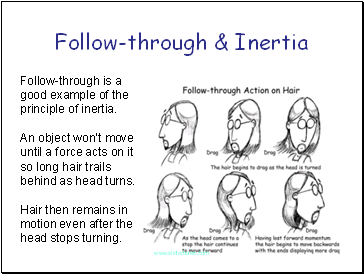## Follow-through & Inertia

Follow-through is a good example of the principle of inertia.

An object won’t move until a force acts on it so long hair trails behind as head turns.

Hair then remains in motion even after the head stops turning.

Slide 16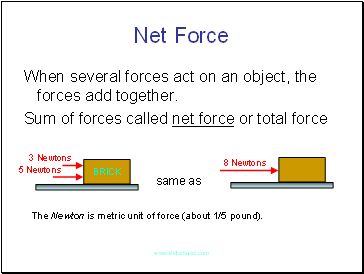## Net Force

same as

The Newton is metric unit of force (about 1/5 pound).

When several forces act on an object, the forces add together.

Sum of forces called net force or total force

BRICK

3 Newtons

5 Newtons

8 Newtons

Slide 17## Equilibrium Rule

same as

If an object is at rest then the net force must be zero. Similarly if in uniform motion.

BRICK

3 Newtons

3 Newtons

Zero Newtons

(No Force)

When this happens we say that forces “balance.”

Slide 18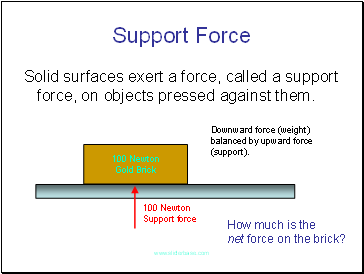## Support Force

Go to page:
1  2  3  4  5  6  7